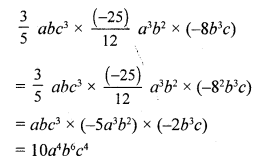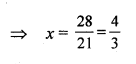# RS Aggarwal Class 7 Solutions Chapter 7 Linear Equations in One Variable CCE Test Paper

In this chapter, we provide RS Aggarwal Solutions for Class 7 Chapter 7 Linear Equations in One Variable CCE Test Paper for English medium students, Which will very helpful for every student in their exams. Students can download the latest RS Aggarwal Solutions for Class 7 Chapter 7 Linear Equations in One Variable CCE Test Paper Maths pdf, free RS Aggarwal Solutions Class 7 Chapter 7 Linear Equations in One Variable CCE Test Paper Maths book pdf download. Now you will get step by step solution to each question.

### RS Aggarwal Solutions for Class 7 Chapter 7 Linear Equations in One Variable CCE Test Paper Download PDF

Question 1.
Solution:
We have:
x3 + y3 + z3 – 3xyz
= (-2)3 + (-1)3 + (3)3 – 3 x (-2) x (-1) x 3
= – 8 – 1 + 27 – 18 = -27 + 27 = 0

Question 2.
Solution:
Co-efficient of x in the given numbers are
(i) -5y
(ii) 2yz
(iii) −32 ab

Question 3.
Solution:
We have:
(4xy – 5x2 – y2 + 6) – (x2 – 2xy + 5y2 – 4)
= 4xy – 5x2 – y2 + 6 – x2 + 2xy – 5y2 + 4
= -6x2 – 6y2 + 6xy +10
= -2 (3x2 + 3y – 3xy – 5)

Question 4.
Solution:
We have:
(2x2 – 3y2 + xy) – (x2 – 2xy + 3y2)
= 2x2 – 3y2 +xy – x2 + 2xy – 3y2
= 2x2 – x2 – 3y2 – 3y2 + xy + 2xy
= x2 – 6y2 + 3xy
x2 – 2xy + 3y2 is less than 2x2 – 3y2 + xy by x2 – 6y + 3xy.

Question 5.
Solution:
We have:Question 6.
Solution:
We have:
(3a + 4) (2a – 3) + (5a – 4) (a + 2)
= {3a (2a – 3) + 4 (2a – 3)} + {5a (a + 2) – 4 (a + 2)}
= (6a2 – 9a + 8a – 12) + (5a2 + 10a – 4a – 8)
= (6a2 – a – 12) + (5a2 + 6a – 8)
= (11a2 + 5a – 20)

Question 7.
Solution:Question 8.
Solution:
We have:Question 9.
Solution:
Let the consecutive odd number be
x and (x + 2)
x + (x + 2) = 68
2x + 2 = 68
2x = 68 – 2 = 66
x = 33
The required numbers are 33 and (33 + 2), i.e., 35.

Question 10.
Solution:
Let Reenu’s present age be x
Then, her father’s present age will be 3x
Reenu’s age after 12 years = (x + 12)
3x + 12 = 2x + 24
x = 12
Reenu’s present age = x = 12 yrs
And her father’s age = 3x = (3 x 12) = 36 yrs

Mark (✓) against the correct answer in each of the following :
Question 11.
Solution:Question 12.
Solution:Question 13.
Solution:Question 14.
Solution:
(c) 18
Let the number be x.
According to the equation, we have:
4x = x + 54
⇒ 3x = 54
⇒ x = 18

Question 15.
Solution:
(b) 52°
Let the two complementary angles be x° and (90 – x)°.
According to the equation, we have:
x – (90 – x) = 14
⇒ 2x = 104
⇒ x = 52
(90° – x)° = 90° – 52° = 38°
The larger angle is 52°.

Question 16.
Solution:
(c) 32 m
Let the length and breadth of the rectangle be l m and b m, respectively.
According to the questions, we have:
l = 2b ……(i)
2 (l + b) = 96 …..(ii)
Now, 2 (2b+ b) = 96
⇒ 6b = 96
⇒ b = 16
Length = 16 x 2 m = 32 m

Question 17.
Solution:
(b) 12 years
Let the ages of A and B be x and y years respectively,Question 18.
Solution:
(i) -2a2 b is a monomial.
(ii) (a2 – 2b2) is a binomial.
(iii) (a + 2b – 3c) is a trinomial.
(iv) In -5ab, the coefficient of a is -5.
(v) In x2 + 2x – 5, the constant term is -5.

Question 19.
Solution:
(i) False.
The coefficient of x is -1.
(ii) False.
The coefficient of x in – 3.
(iii) False.
LHS = (5x – 7) – (3x – 5) = 5x – 7 – 3x + 5 = 2x – 2.
(iv) True.
LHS = (3x + 5y) (3x – 5y)
= 3x (3x – 5y) + 5y (3x – 5y)
= 9x2 – 15xy + 15xy – 25y2
= 9x2 – 25y2
(v) True
(a2 + b2)All Chapter RS Aggarwal Solutions For Class 7 Maths

—————————————————————————–

All Subject NCERT Exemplar Problems Solutions For Class 7

All Subject NCERT Solutions For Class 7

*************************************************

I think you got complete solutions for this chapter. If You have any queries regarding this chapter, please comment on the below section our subject teacher will answer you. We tried our best to give complete solutions so you got good marks in your exam.

If these solutions have helped you, you can also share rsaggarwalsolutions.in to your friends.